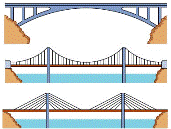## Civil and Environmental Engineering## Civil and Environmental Engineering Faculty Publications

Hoshin V. Gupta

Uwe Ehret

6-11-2021

#### Citation

Gupta, H.V.; Ehsani, M.R.; Roy, T.; Sans-Fuentes, M.A.; Ehret, U.; Behrangi, A. Computing Accurate Probabilistic Estimates of One-D Entropy from Equiprobable Random Samples. Entropy 2021, 23, 740. https://doi.org/10.3390/e23060740

CC-BY

#### Abstract

We develop a simple Quantile Spacing (QS) method for accurate probabilistic estimation of one-dimensional entropy from equiprobable random samples, and compare it with the popular Bin-Counting (BC) and Kernel Density (KD) methods. In contrast to BC, which uses equal-width bins with varying probability mass, the QS method uses estimates of the quantiles that divide the support of the data generating probability density function (pdf) into equal-probability-mass intervals. And, whereas BC and KD each require optimal tuning of a hyper-parameter whose value varies with sample size and shape of the pdf, QS only requires specification of the number of quantiles to be used. Results indicate, for the class of distributions tested, that the optimal number of quantiles is a fixed fraction of the sample size (empirically determined to be ∼0.25–0.35), and that this value is relatively insensitive to distributional form or sample size. This provides a clear advantage over BC and KD since hyper-parameter tuning is not required. Further, unlike KD, there is no need to select an appropriate kernel-type, and so QS is applicable to pdfs of arbitrary shape, including those with discontinuous slope and/or magnitude. Bootstrapping is used to approximate the sampling variability distribution of the resulting entropy estimate, and is shown to accurately reflect the true uncertainty. For the four distributional forms studied (Gaussian, Log-Normal, Exponential and Bimodal Gaussian Mixture), expected estimation bias is less than 1% and uncertainty is low even for samples of as few as 100 data points; in contrast, for KD the small sample bias can be as large as −10% and for BC as large as −50%. We speculate that estimating quantile locations, rather than bin-probabilities, results in more efficient use of the information in the data to approximate the underlying shape of an unknown data generating pdf.

COinS